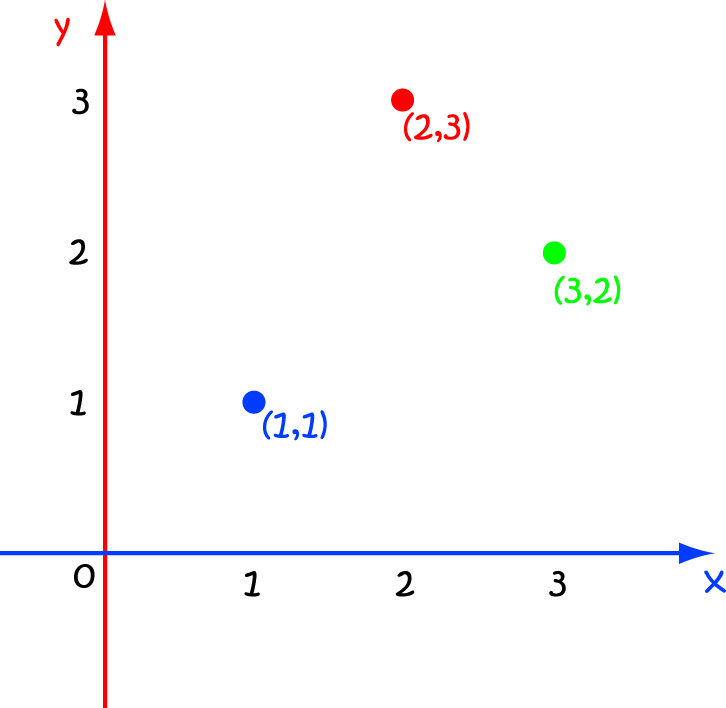# Definition of PlotTo plot something is to draw it, or put it into position on a graph.

We often talk about plotting points in mathematics.

In the picture, the points $(1,1)$,$(2,3)$ and $(3,2)$ have been plotted.

### Description

The aim of this dictionary is to provide definitions to common mathematical terms. Students learn a new math skill every week at school, sometimes just before they start a new skill, if they want to look at what a specific term means, this is where this dictionary will become handy and a go-to guide for a student.

### Audience

Year 1 to Year 12 students

### Learning Objectives

Learn common math terms starting with letter P

Author: Subject Coach
You must be logged in as Student to ask a Question.